Tamilnadu State Board New Syllabus Samacheer Kalvi 12th Computer Science Guide Pdf Chapter 4 Algorithmic Strategies Text Book Back Questions and Answers, Notes.

## Tamilnadu Samacheer Kalvi 12th Computer Science Solutions Chapter 4 Algorithmic Strategies

### 12th Computer Science Guide Algorithmic Strategies Text Book Questions and Answers

I. Choose the best answer (1 Mark)

Question 1.
The word comes from the name of a Persian mathematician Abu Ja’far Mohammed ibn-i Musa al Khwarizmi is called?
a) Flowchart
b) Flow
c) Algorithm
d) Syntax
c) Algorithm

Question 2.
From the following sorting algorithms which algorithm needs the minimum number of swaps?
a) Bubble sort
b) Quick sort
c) Merge sort
d) Selection sort
d) Selection sortQuestion 3.
Two main measures for the efficiency of an algorithm are
a) Processor and memory
b) Complexity and capacity
c) Time and space
d) Data and space
c) Time and space

Question 4.
The complexity of linear search algorithm is
a) O(n)
b) O(log n)
c) O(n2)
d) O(n log n)
a) O(n)Question 5.
From the following sorting algorithms which has the lowest worst-case complexity?
a) Bubble sort
b) Quick sort
c) Merge sort
d) Selection sort
c) Merge sort

Question 6.
Which of the following is not a stable sorting algorithm?
a) Insertion sort
b) Selection sort
c) Bubble sort
d) Merge sort
b) Selection sort

Question 7.
Time Complexity of bubble sort in best case is
a) θ (n)
b) θ (nlogn)
c) θ (n2)
d) θ n(logn)2)
a) θ (n)Question 8.
The Θ notation in asymptotic evaluation represents
a) Base case
b) Average case
c) Worst case
d) NULL case
b) Average case

Question 9.
If a problem can be broken into sub problems which are reused several times, the problem possesses which property?
a) Overlapping sub problems
b) Optimal substructure
c) Memoization
d) Greedy
a) Overlapping sub problemsQuestion 10.
In dynamic programming, the technique of storing the previously calculated values is called ?
a) Saving value property
b) Storing value property
c) Memoization
d) Mapping
c) Memoization

II. Answer the following questions (2 Marks)

Question 1.
What is an Algorithm?
An algorithm is a finite set of instructions to accomplish a particular task. It is a step-by-step procedure for solving a given problem. An algorithm can be implemented in any suitable programming language.

Question 2.
Define Pseudocode

• Pseudocode is a notation similar to programming languages.
• Pseudocode is a mix of programming-language-like constructs and plain English.Question 3.
Who is an Algorist?
A person skilled in the design of algorithms is called Agorist.

Question 4.
What is Sorting?
Sorting is a method of arranging a group of items in ascending or descending order. Various sorting techniques in algorithms are Bubble Sort, Quick Sort, Heap Sort, Selection Sort, Insertion Sort.

Question 5.
What is searching? Write its types.

1. Searching is a process of finding a particular element present in given set of elements.
2. Some of the searching types are:

Linear Search
Binary Search.III. Answer the following questions (3 Marks)

Question 1.
List the characteristics of an algorithm
Input, Output, Finiteness, Definiteness, Effectiveness, Correctness, Simplicity, Unambiguous, Feasibility, Portable and Independent.

Question 2.
Discuss Algorithmic complexity and its types

• The complexity of an algorithm f (n) gives the running time and/or the storage space required by the algorithm in terms of n as the size of input data.
• Time Complexity: The Time complexity of an algorithm is given by the number of steps taken by the algorithm to complete the process.
• Space Complexity: Space complexity of an algorithm is the amount of memory required to run to its completion.

The space required by an algorithm is equal to the sum of the following two components:

• A fixed part is defined as the total space required to store certain data and variables for an algorithm.
• Example: simple variables and constants used in an algorithm.
• A variable part is defined as the total space required by variables, which sizes depends on the problem and its iteration.
• Example: recursion used to calculate factorial of a given value n.Question 3.
What are the factors that influence time and space complexity?
Time Complexity:
The Time complexity of an algorithm is given by the number of steps taken by the algorithm to complete the process.

Space Complexity:
Space complexity of an algorithm is the amount of memory required to run to its completion. The space required by an algorithm is equal to the sum of the following two components:

A fixed part is defined as the total space required to store certain data and variables for an algorithm. For example, simple variables and constants used in an algorithm. A variable part is defined as the total space required by variables, which sizes depends on the problem and its iteration. For example, recursion used to calculate the factorial of a given value n.

Question 4.
Write a note on Asymptotic notation

• Asymptotic Notations are languages that use meaningful statements about time and space complexity.
• The following three asymptotic notations are mostly used to represent time complexity of algorithms:

Big O:
Big O is often used to describe the worst -case of an algorithm.

Big Ω:

• Big O mega is the reverse Big O if Bi O is used to describe the upper bound (worst – case) of an asymptotic function,
• Big O mega is used to describe the lower bound (best-case).

Big Θ:
When an algorithm has complexity with lower bound = upper bound, say that an algorithm has a complexity 0 (n log n) and Ω (n log n), it actually has the complexity Θ (n log n), which means the running time of that algorithm always falls in n log n in the best-case and worst-case.Question 5.
What do you understand by Dynamic programming?
Dynamic programming is an algorithmic design method that can be used when the solution to a problem can be viewed as the result of a sequence of decisions. The dynamic programming approach is similar to divide and conquer. The given problem is divided into smaller and yet smaller possible subproblems.

IV. Answer the following questions (5 Marks)

Question 1.
Explain the characteristics of an algorithm.

 Input Zero or more quantities to be supplied. Output At least one quantity is produced. Finiteness Algorithms must terminate after a finite number of steps. Definiteness all operations should be well defined. For example, operations involving division by zero or taking square root for negative numbers are unacceptable. Effectiveness Every instruction must be carried out effectively. Correctness The algorithms should be error-free. Simplicity Easy to implement. Unambiguous The algorithm should be clear and unambiguous. Each of its steps and their inputs/outputs should be clear and must lead to only one meaning. Feasibility Should be feasible with the available resources. Portable An algorithm should be generic, independent of any programming language or an operating system able to handle all range of inputs. Independent An algorithm should have step-by-step directions, which should be independent of any programming code.Question 2.
Discuss Linear search algorithm

• Linear search also called sequential search is a sequential method for finding a particular value in a list.
• This method checks the search element with each element in sequence until the desired element is found or the list is exhausted. In this searching algorithm, list need not be ordered.

Pseudocode:

• Traverse the array using for loop
• In every iteration, compare the target search key value with the current value of the list.
• If the values match, display the current index and value of the array.
• If the values do not match, move on to the next array element.
• If no match is found, display the search element not found.

Example: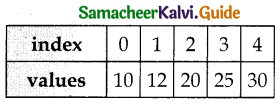• To search the number 25 in the array given below, a linear search will go step by step in a sequential order starting from the first element in the given array if the search element is found that index is returned otherwise the search is continued till the last index of the array.
• In this example number 25 is found at index number 3.

Question 3.
What is Binary search? Discuss with example
Binary Search:

• Binary search also called a half-interval search algorithm.
• It finds the position of a search element within a sorted array.
• The binary search algorithm can be done as a dividend- and -conquer search algorithm and executes in logarithmic time.

Example:

• List of elements in an array must be sorted first for Binary search. The following example describes the step by step operation of binary search.
• Consider the following array of elements, the array is being sorted so it enables to do the binary search algorithm.
Let us assume that the search element is 60 and we need to search the location or index of search element 60 using binary search.• First ‘we find index of middle element of the array by using this formula:
mid = low + (high – low)/2
• Here it is, 0+(9 – 0)/2 = 4 (fractional part ignored)v So, 4 is the mid value of the array.• Now compare the search element with the value stored at mid-value location 4. The value stored at location or index 4 is 50, which is not match with search element. As the search value 60 is greater than 50.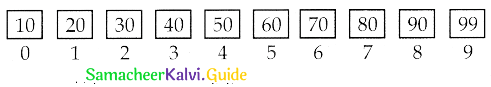• Now we change our low to mid + land find the new mid-value again using the formula.
low to mid + 1
mid = low + (high -low) / 2
• Our new mid is 7 now. We compare the value stored at location 7 with our target value 31.• The value stored at location or index 7 is not a match with search element, rather it is more than what we are looking for. So, the search element must be in the lower part from the current mid-value location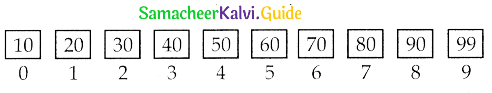• The search element still not found. Hence, we calculated the mid again by using the formula.
high = mid – 1
mid = low + (high – low)/2
Now the mid-value is 5.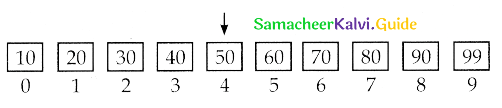• Now we compare the value stored at location 5 with our search element. We found that it is a match.• We can conclude that the search element 60 is found at the location or index 5.
• For example, if we take the search element as 95, For this value this binary search algorithm returns the unsuccessful result.Question 4.
Explain the Bubble sort algorithm with example
Bubble sort:

• Bubble sort algorithm simple sorting algorithm.
• The algorithm starts at the beginning of the I list of values stored in an array.
• It compares each pair of adjacent elements and swaps them if they are in the unsorted order.
• This comparison and passed to be continued until no swaps are needed, which indicates that the list of values stored in an array is sorted.
• The algorithm is a comparison sort, is named for the way smaller elements “bubble” to the top of the list.
• Although the algorithm is simple, it is too slow and less efficient when compared to insertion sort and other sorting methods.
• Assume list is an array of n elements. The swap function swaps the values of the given array elements.

Pseudocode:

• Start with the first element i.e., index = 0, compare the current element with the next element of the array.
• If the current element is greater than the next element of the array, swap them.
If the current element is less than the next or right side of the element, move to the next element. Go to Step 1 and repeat until the end of the index is reached.

Let’s consider an array with values {15, 11, 16, 12, 14, 13} Below, we have a pictorial representation of how bubble sort will sort the given array.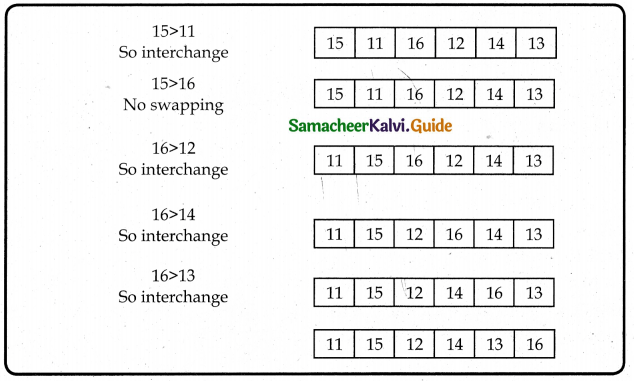The above pictorial example is for iteration-d. Similarly, remaining iteration can be done. The final iteration will give the sorted array. At the end of all the iterations we will get the sorted values in an array as given below: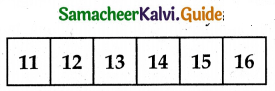Question 5.
Explain the concept of dynamic programming with a suitable example.
Dynamic programming:
Dynamic programming is an algorithmic design method that can be used when the solution to a problem can be viewed as the result of a sequence of decisions. The dynamic programming approach is similar to divide and conquer. The given problem is divided into smaller and yet smaller possible subproblems.

Dynamic programming is used whenever problems can be divided into similar sub-problems so that their results can be re-used to complete the process. Dynamic programming approaches are used to find the solution in an optimized way. For every inner subproblem, the dynamic algorithm will try to check the results of the previously solved sub-problems. The solutions of overlapped subproblems are combined in order to get a better solution.

Steps to do Dynamic programming:

1. The given problem will be divided into smaller overlapping sub-problems.
2. An optimum solution for the given problem can be achieved by using the result of a smaller sub-problem.
3. Dynamic algorithms use Memoization.

Fibonacci Series – An example:
Fibonacci series generates the subsequent number by adding two previous numbers. Fibonacci series starts from two numbers – Fib 0 & Fib 1. The initial values of fib 0 & fib 1 can be taken as 0 and 1.
Fibonacci series satisfies the following conditions:
Fibn = Fibn-1 + Fibn-2
Hence, a Fibonacci series for the n value 8 can look like this
Fib8 = 0 1 1 2 3 5 8 13

Fibonacci Iterative Algorithm with Dynamic programming approach:
The following example shows a simple Dynamic programming approach for the generation of the Fibonacci series.
Initialize f0 = 0, f1 = 1
step – 1: Print the initial values of Fibonacci f0 and f1
step – 2: Calculate fibanocci fib ← f0 + f1
step – 3: Assign f0 ← f1, f1 ← fib
step – 4: Print the next consecutive value of Fibonacci fib
step – 5: Go to step – 2 and repeat until the specified number of terms generated
For example, if we generate Fibonacci series up to 10 digits, the algorithm will generate the series as shown below:
The Fibonacci series is: 0 1 1 2 3 5 8 1 3 2 1 3 4 5 5Choose the best answer: (1 Marks)

Question 1.
Which one of the following is not a data structure?
(a) Array
(b) Structures
(c) List, tuples
(d) Database
(d) Database

Question 2.
Linear search is also called
a) Quick search
b) Sequential search
c) Binary search
d) Selection search
b) Sequential searchQuestion 3.
Which is a wrong fact about the algorithm?
(a) It should be feasible
(b) Easy to implement
(c) It should be independent of any programming languages
(d) It should be generic
(c) It should be independent of any programming languages

Question 4.
Which search algorithm can be done as divide and- conquer search algorithm?
a) linear
b) Binary search
c) Sequential
d) Bubble
b ) Binary searchQuestion 5.
Which of the following sorting algorithm is too slow and less efficient?
a) Selection
b) Bubble
c) Quick
d) Merge
b) Bubble

Question 6.
Which of the following programming is used whenever problems can be divided into similar sub-problems?
a) Object-oriented
b) Dynamic
c) Modular
d) Procedural
b) DynamicQuestion 7.
Which of the following is the reverse of Big O?
a) Big μμ
b) Big Ω
c) Big symbol
d) Big ΩΩ
b) Big Ω

Question 8.
How many different phases are there in the analysis of algorithms and performance evaluations?
(a) 1
(b) 2
(c) 3
(d) Many
(b) 2Question 9.
Which of the following is a finite set of instructions to accomplish a particular task?
a) Flowchart
b) Algorithm
c) Functions
d) Abstraction
b) Algorithm

Question 10.
The way of defining an algorithm is called
a) Pseudo strategy
b) Programmatic strategy
c) Data structured strategy
d) Algorithmic strategy
d) Algorithmic strategy

Question 11.
Time is measured by counting the number of key operations like comparisons in the sorting algorithm. This is called as ……………………………
(a) Space Factor
(b) Key Factor
(c) Priori Factor
(d) Time Factor
(d) Time FactorQuestion 12.
Efficiency of an algorithm decided by
a) Definiteness, portability
b) Time, Space
c) Priori, Postriori
d) Input/output
b) Time, Space

Question 13.
Which of the following should be written for the selected programming language with specific syntax?
a) Algorithm
b) Pseudocode
c) Program
d) ProcessQuestion 14.
How many components required to find the space required by an algorithm?
a) 4
b) 3
c) 2
d) 6
c) 2

Question 15.
Which of the following component is defined as the total space required to store certain data and variables for an algorithm?
a) Time part
b) Variable part
c) Memory part
d) Fixed part
d) Fixed part

Question 16.
Which is true related to the efficiency of an algorithm?
(I) Less time, more storage space
(II) More time, very little space
(a) I is correct
(b) II is correct
(c) Both are correct
(d) Both are wrong
(c) Both are correctQuestion 17.
Time and Space complexity could be considered for an
a) Algorithmic strategy
b) Algorithmic analysis
c) Algorithmic efficiency
d) Algorithmic solution
c) Algorithmic efficiency

Question 18.
Which one of the following is not an Asymptotic notation?
(a) Big
(b) Big $$\Theta$$
(c) Big Ω
(d) Big ⊗
(d) Big ⊗Question 19.
How many asymptotic notations are mostly used to represent time complexity of algorithms?
a) Two
b) Three
c) One
d) Many
b) Three

II. Answer the following questions (2 and 3 Marks)

Question 1.
Define fixed part in the space complexity?
A fixed part is defined as the total space required to store certain data and variables for an algorithm. For example, simple variables and constants used in an algorithm.

Question 2.
Write a pseudo code for linear search

• Traverse the array using ‘for loop’
• In every iteration, compare the target search key value with the current value of the list.
• If the values match, display the current index and value of the array
• If the values do not match, move on to the next array element
• If no match is found, display the search element not found.Question 3.
Design an algorithm to find the square of the given number and display the result?
Problem: Design an algorithm to find the square of the given number and display the result. The algorithm can be written as:

• Step 1 – start the process
• Step 2 – get the input x
• Step 3 – calculate the square by multiplying the input value ie., square ← x* x
• Step 4 – display the resulting square
• Step 5 – stop

The algorithm could be designed to get a solution of a given problem. A problem can be solved in many ways. Among many algorithms, the optimistic one can be taken for implementation.Question 4.
Write a pseudo code for bubble sort

• Start with the first element i.e., index = 0, compare the current element with the next element of the array.
• If the current element is greater than the next element of the array, swap them.
• If the current element is less than the next or right side of the element, move to the next element.
• Go to Step 1 and repeat until end of the index is reached.Question 5.
Write a pseudo code for selection sort.

• Start from the first element i.e., index= 0, we search the smallest element in the array, and replace it with the element in the first position.
• Now we move on to the second element position, and look for smallest element present in the sub-array, from starting index to till the last index of sub-array.
• Now replace the second smallest identified in step-2 at the second position in the or original array, or also called first position in the sub-array.

Question 6.
Write a note on Big omega asymptotic notation

• Big Omega is the reverse Big 0, if Big 0 is used to describe the upper bound (worst – case) of a asymptotic function,
• Big Omega is used to describe the lower bound (best-case).Question 7.
Name the factors where the program execution time depends on?
The program execution time depends on:

1. Speed of the machine
2. Compiler and other system Software tools
3. Operating System
4. Programming language used
5. The volume of data required

Question 8.
Write a note on memoization.
Memoization or memoisation is an optimization technique used primarily to speed up computer programs by storing the results of expensive function calls and returning the cached result when the same inputs occur again.

Question 9.
Give examples of data structures.
Examples for data structures are arrays, structures, list, tuples, dictionary.

Question 10.
Define- Algorithmic Strategy?
The way of defining an algorithm is called Algorithmic Strategy.

Question 11.
Define algorithmic solution?
An algorithm that yields expected output for a valid input is called an algorithmic solutionQuestion 12.
Define- Algorithm Analysis?
An estimation of the time and space complexities of an algorithm for varying input sizes is called Algorithm Analysis.

Question 13.
What are the different phases in the analysis of algorithms and performance?
Analysis of algorithms and performance evaluation can be divided into two different phases:
A Priori estimates: This is a theoretical performance analysis of an algorithm. Efficiency of an algorithm is measured by assuming the external factors.
Posteriori testing: This is called performance measurement. In this analysis, actual statistics like running time and required for the algorithm executions are collected.Question 14.
Define the Best algorithm?
The best algorithm to solve a given problem is one that requires less space in memory and takes less time to execute its instructions to generate output.

Question 15.
Write a note Asymptotic Notations?